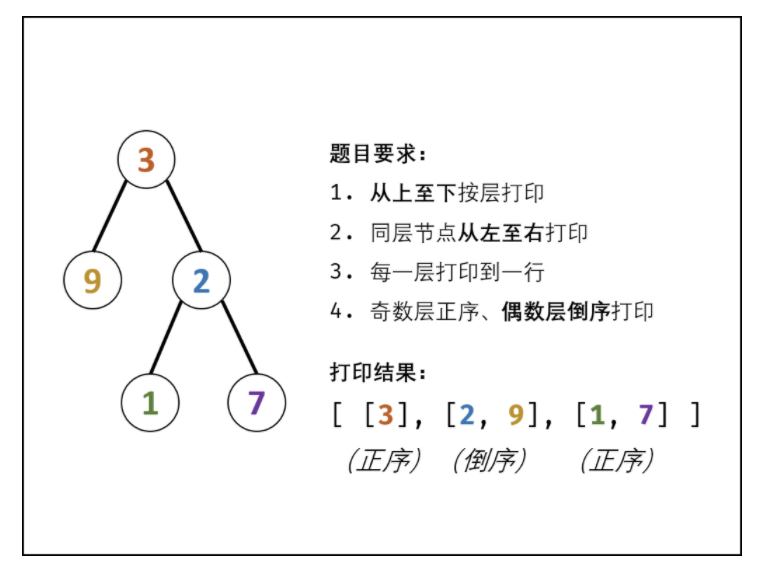# 算法攻关 - 重上到下打印二叉树 (O(n))_offer323

/ \

9 20

/ \

15 7

[

,

[20,9],

[15,7]

]

# 二、思路

• 奇数层 则添加至 tmp 尾部 ，

• 偶数层 则添加至 tmp 头部 。##### 算法流程：
1. 特例处理： 当树的根节点为空，则直接返回空列表 [] ；

2. 初始化： 打印结果空列表 res ，包含根节点的双端队列 deque ；

3. BFS 循环： 循环打印奇 / 偶数层，当 deque 为空时跳出；

1. 返回值： 返回打印结果列表 res 即可；

• 时间复杂度 O(N)

• 空间复杂度 O(N)

# 三、代码实现

class TreeNode{        int val;        TreeNode left;        TreeNode right;        TreeNode(int x){            this.val =x;        }    }    public List<List<Integer>> levelOrder(TreeNode root) {        Deque<TreeNode> deque = new LinkedList<>();        List<List<Integer>> res = new ArrayList<>();        if(root != null) deque.add(root);        while(!deque.isEmpty()) {            // 打印奇数层            List<Integer> tmp = new ArrayList<>();            for(int i = deque.size(); i > 0; i--) {                // 从左向右打印                TreeNode node = deque.removeFirst();                tmp.add(node.val);                // 先左后右加入下层节点                if(node.left != null) deque.addLast(node.left);                if(node.right != null) deque.addLast(node.right);            }            res.add(tmp);            if(deque.isEmpty()) break; // 若为空则提前跳出            // 打印偶数层            tmp = new ArrayList<>();            for(int i = deque.size(); i > 0; i--) {                // 从右向左打印                TreeNode node = deque.removeLast();                tmp.add(node.val);                // 先右后左加入下层节点                if(node.right != null) deque.addFirst(node.right);                if(node.left != null) deque.addFirst(node.left);            }            res.add(tmp);        }        return res;    }

# 四、小结## 评论